### ESTIMATION OF MASS IN CORONAL MASS EJECTIONS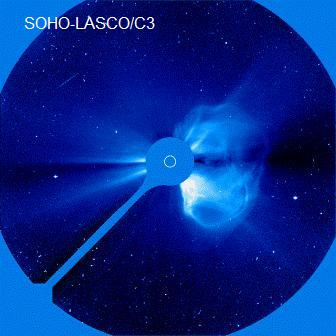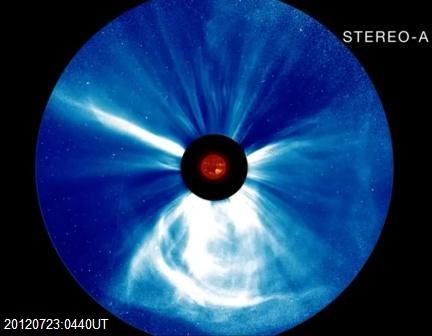Left image: CME as observed in white light by the LASCO C3 coronagraph on the SOHO spacecraft at the Earth Lagrange point [NASA/SOHO]
Right image: CME as observed in white light by the coronagraph on the STEREO-A spacecraft in heliocentric orbit at 1 AU [NASA/STEREO]

CORONAL MASS EJECTIONS

The term coronal mass ejection can refer to the process whereby a large quantity of material is ejected from the solar corona into the interplanetary medium or it can refer to the cloud of ejected matter. It is the latter definition that is generally meant when the abbreviation CME is used.

CME's have been studied seriously since around 1970 when the first coronagraphs were lofted above the Earth's atmosphere. One of the CME parameters of most interest is the amount of material or mass carried in the CME. Estimates of this mass are now routinely carried out by the use of photometry whereby the integrated brightness of the CME is measured and used to calculate the mass. This method will be described below.

Following this a simple geometric method will be presented which can be used to give a rough order of magnitude estimate of CME mass.

CME PHOTOMETRY

A CME is visible in white light due to scattering of solar photospheric light by free electrons in the CME plasma. The brighter the CME, the greater the number of free electrons and thus the greater the number of positive ions and mass of the plasma.

The standard method to determine the mass of a CME is by photometry of a white-light coronagraph image of the CME. The steps in this process are:

1. Obtain the coronagraph image of the CME.
2. Obtain an identical coronagraph image without the CME.
3. Subtract image 2 from image 1 leaving only the light from the CME.
4.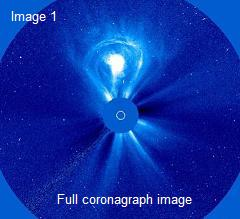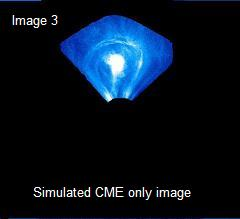5. Calibrate image 3 into appropriate units.
6. Measure the light intensity of each pixel in the image. The value will be a function of the position angle of the CME to the plane of the sky (theta) and the elongation of the pixel from the Sun (epsilon).
7. For each pixel calculate the intensity due to a single electron. The intensity is due to Thomson scattering of photospheric light by free electrons in the CME plasma. Assume 100% ionisation. This intensity will also be a function of theta and epsilon.
8. Divide 5 by 6 giving the number of electrons in each CME pixel.
9. Multiply 7 by the average mass of each ion in the CME plasma. It is normally assumed that the plasma is 90% H and 10% He.
10. Sum the pixel masses over the whole image to get total CME mass.
These calculations are shown in detail in the figure below.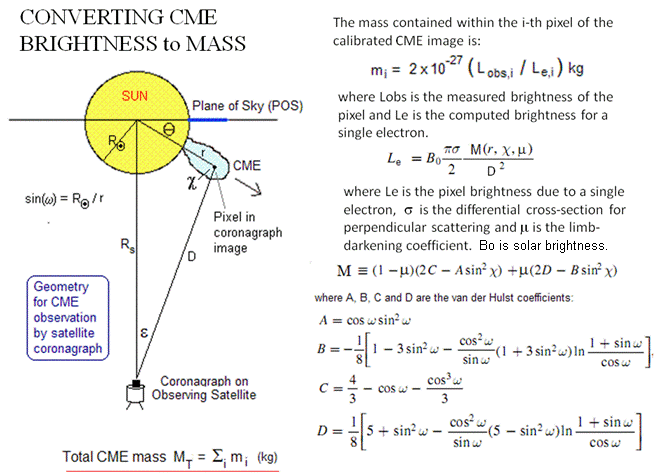The coronagraph image calibration together with the above calculations are normally carried out using software tools available from the organisation that supplies the coronagraph images. For the LASCO images there is an analysis package called 'LASCO Solarsoft' that is available from NASA Goddard.

A SIMPLE GEOMETRIC MASS CALCULATION

It is also possible to perform a rough order of magnitude estimation of a CME mass using only geometric ideas together with a knowledge of coronal particle density near Earth orbit (but outside the Earth's magnetosphere).

The details on how to do this are shown in the figure below. Note that the CME illustrated here is a very large CME. However, the calculations can be applied to any size CME. Although we can look at a coronagraph image and estimate the CME size, we do not have an estimate for the CME particle density just after ejection from the Sun. It is thus necessary for us to use a simple scaling relation to compute the CME size near Earth orbit < Coronal Mass Ejections >.

Note that the sizes shown in this diagram (Sun, CME in both positions and distance from Sun to Earth are roughly to scale.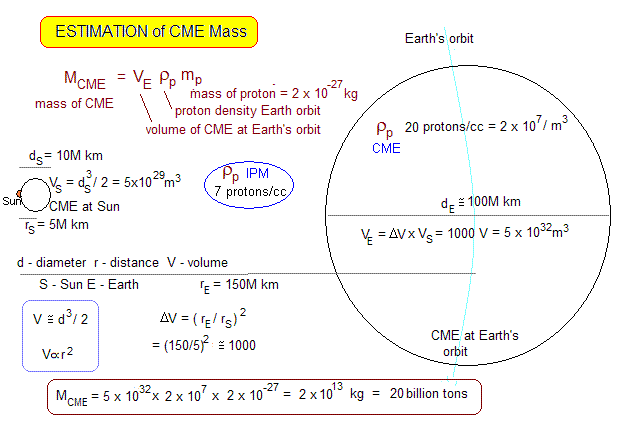REFERENCES

• Vourlidas & Howard, Ap J, 642:1216-1221 (2006)
• Koning, ApJ, 844:61-74 (2017)Australian Space Academy